# Future of libraries

You know that thanks to the Internet, electronic communications and developing online resources on the Internet annually declining number of traditional readership by 17%. These deal is irreversible evolutionary shift from old books from libraries to immediately available and cheaper information from the Internet.

The library had in 1991 3336 readers. Determine the year in which count of readers will be only 289.

Result

x =  2004

#### Solution:Leave us a comment of example and its solution (i.e. if it is still somewhat unclear...):

Showing 0 comments:Be the first to comment!#### To solve this verbal math problem are needed these knowledge from mathematics:

Our percentage calculator will help you quickly calculate various typical tasks with percentages.

## Next similar examples:

1. Gp - 80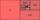Sum of the first four members of a geometric progression is 80. Determine they if we know that the fourth member is nine times greater than the second.
2. A perineumA perineum string is 10% shorter than its original string. The first string is 24, what is the 9th string or term?
3. JUDr. Usury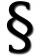Judge JUDr. Usury approved the agreement on guilt and punishment where confessed to pay daily interest 0.18%. How big is a yearly interest? Year has 360 days.
4. GP - 8 itemsDetermine the first eight members of a geometric progression if a9=512, q=2
5. Six termsFind the first six terms of the sequence a1 = -3, an = 2 * an-1
6. Geometric progression 2There is geometric sequence with a1=5.7 and quotient q=-2.5. Calculate a17.
7. Geometric sequence 4It is given geometric sequence a3 = 7 and a12 = 3. Calculate s23 (= sum of the first 23 members of the sequence).
8. Five membersWrite first 5 members geometric sequence and determine whether it is increasing or decreasing: a1 = 3 q = -2
9. Theorem proveWe want to prove the sentence: If the natural number n is divisible by six, then n is divisible by three. From what assumption we started?
10. Tenth memberCalculate the tenth member of geometric sequence when given: a1=1/2 and q=2
11. UV radiation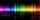The glass of 1 mm thickness absorb 4% of UV radiation passing through. How many percent of UV rays absorb glass with a thickness 1.1 cm, made from 1 mm thick those glasses?
12. Piano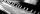If Suzan practicing 10 minutes at Monday; every other day she wants to practice 2 times as much as the previous day, how many hours and minutes will have to practice on Friday?
13. Geometric progression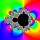Fill 4 numbers between 4 and -12500 to form geometric progression.
14. Gain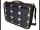Find the gain whenever the output power is 21x the input power.
15. GP membersThe geometric sequence has 10 members. The last two members are 2 and -1. Which member is -1/16?
16. PopulationThe town has 65,000 inhabitants. 40 years ago there were 157,000. How many people will live in town in 10 years if the average rate in population is as in previous years?
17. SequenceFind the common ratio of the sequence -3, -1.5, -0.75, -0.375, -0.1875. Ratio write as decimal number rounded to tenth.